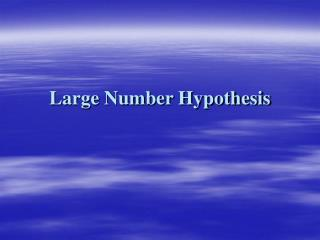Download PresentationLarge Number HypothesisLarge Number Hypothesis - PowerPoint PPT Presentation

Download PresentationLarge Number Hypothesis
An Image/Link below is provided (as is) to download presentation

Download Policy: Content on the Website is provided to you AS IS for your information and personal use and may not be sold / licensed / shared on other websites without getting consent from its author. While downloading, if for some reason you are not able to download a presentation, the publisher may have deleted the file from their server.

- - - - - - - - - - - - - - - - - - - - - - - - - - - E N D - - - - - - - - - - - - - - - - - - - - - - - - - - -
Presentation Transcript

1. Large Number Hypothesis

2. Universal Constants • In physics, many numbers that we believe do not change with time, they are “universal constants”. • 4 so-called universal constants of nature: • speed of light, c • Gravitational constant, G • Planck constant, h • Boltzmann constant, k • appear unchanged over time • valid throughout the entire universe

3. Speed of light, c • c = 3.00 x 108ms-1 • Light is an electromagnetic wave, the speed of light is a constant. • When light travels through a medium, e.g., glass, its speed is reduced according to the density of the glass. Speed of light in air = c Speed in medium (eg. glass) < c

4. Gravitational constant, G • Any two objects attract each other with a force proportional to the product of their masses divided by the square of their separation. • The constant of proportionality, called G, is what is known as the Gravitation constant. • G = 6.67 x 10-11Nm2kg-2 • Einstein's theory of General Relativity reinterprets the meaning of G in terms of the curvature of space-time around the objects.

5. Planck constant, h • Planck’s theory of quantized energy, which we now called quantum theory. • Electromagnetic radiation was not just a continuous wave, radiations are emitted or absorbed in discrete units called “quanta”. • The energy of these quanta is proportional to the frequency of the radiation. The constant of proportionality called Planck constant. • h = 6.63 x 10-34 Js

6. Boltzmann constant, k • The product of the pressure and volume is proportional to the number of molecules times the temperature. • The constant of proportionality is known as the Boltzmann constant k. • k = 1.38 x 10-23 JK-1

7. Large Number Hypothesis（LNH） • It was first conceived and coined by the famous physicist Paul Dirac 。 • Several very large dimensionless numbers of the order ~ 「1039」are constructible from certain physical parameters of nature. • Example： The ratio of the Coulomb force (the force between electromagnetic charges) to the gravitational force (the force between masses in the universe) for a proton-electron pair is of the order of 1039.

8. An example for contrast, the ratio of the Planck constant and the electric charge is about 137. • Dirac believes these large dimensionless numbers are somewhat related to each other, e.g., a relationship exists between cosmological and microscopic processes. The ratio of the gravitational and the coulomb forces is not a constant, but increases proportionally with the age of the universe. Are the universal constants (G、c、h、k)is not constant?!

9. Value of G • Value of G which differs from the previous accepted value by 0.1% to 0.7%. • Two possibilities exist: 1. we underestimated the uncertainty in our measurement of G; 2. G actually varies with time. • Einstein's theory of relativity requires G to be a constant. →This theory have to be modified if G varies with time!

10. Fine Structure Constant • the fine structure constant is a combination of electric charge, Planck constant and speed of light. It measures the strength of interactions between charged particles and electromagnetic fields. • Results from spectral analysis of “quasars” indicates that this constant varies with time. • Then, which constant varies with time： the electric charge? the Planck constant? or the speed of light?

11. Amount of Substance in the Universe • Amount of Particles in the universe is estimated to be about 1078, which is equal to the square of the age of the universe. • May the amount of particles in the universe increase with the square of the age of the universe? In other words, may new matter be continually being created?

12. Models of the Universe • 3 different models of the universe: the open, the closed and the stationary model. • The open model：the universe is forever expanding and there will be no end of the universe. The closed model：at some point in the future, the universe will collapse into a point, denoting the end of the universe. The stationary model：the universe neither expands nor contracts, just remain as it is. • From modern observation, the universe is expanding ; • The maximum size of universe predicted by the close model is not supported by the age of the universe； • What remains is the open universe.

13. Conclusion • Dirac conclude that there are two different time evolutions in nature that are closely related to one another: The time evolution which governs the motions of galaxies, planets, and the evolution of the Universe. The time evolution which governs the periodic vibrations of atoms and the atomic time Appear in Einstein's theory of gravitation, or the general theory of relativity. Appears in the theory of atoms, or the quantum theory

14. ~ End ~